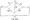# Control Systems January 2009

Note : Answer FIVE full questions, selecting at least TWO questions from each part.

PART-A

1 a. Distinguish between open loop and closed loop control system. Describe two examples for each.

b. Write the differential equations for the mechanical system shown in fig. 1(b) and obtain f -v and f- I analogous electrical circuits. Fig. 1(b)

2  a. Draw a block diagram for the bridge circuit shown in fig.2(a), where vi and io   are the input Io(s) and output variables respectively. Also determine technique. Vi(s) by block diagram reductionb.  For the system represented by the following equation, find the transfer function X(S) U(S) By signal flow graph, x = xi + a 3 U Xj =-piXi +x2+a2U2 =~P2X1 +0ClU

3 a. Considering the response of a second order system to a unit step input, derive the following : i) Peak time (tp) ii) Rise time (tr) iii) Maximum overshoot (Mp).

b.  Assuming the time constant T of the controller to be 3 sec and the ratio of the torque to inertia K/J to be 3 rad2/sec2, find the damping ratio, rise time, peak time and maximum overshoot (Mp) of the system shown in    .c. For a unity feedback system given by G(s) =20(S+2) S(S + 3)(S + 4)

i)     Find the static error constant

ii)    Find the steady state error for r(t) – 3 u(t) + 5(t) u(t).

4  a. What are the difficulties encountered while assessing the R-H criteria and how do you eliminate these difficulties, explain with examples.

b. The open loop transfer function of a unity feedback control system is given by

G(S)  . Apply R-H criteria. Show that the system is unstable. Confirm S(1 + 0.05S)(1 + 0.2S) that the introduction of the two terminal pair network connected in cascade with G(S) makes the system stable. Ci – 0.5jliF C2 = IOjiF Ri – 1MQ.

c. Ascertain the stability of the system given by the characteristic equation S6 + 3 S5 + 5 S4 + 9 S3 + 8 S2 + 6 S + 4 = 0.

PART -B

5 a. State the different rules for the construction of root locus.

b. Sketch the root locus for the system G(S) H(S) = K S(S + l)(S + 2)(S + 3)

6 a. State and explain Nyquist stability criterion.

b. Draw the complete Nyquist plot of the system whose loop transfer function is given byG(S) en by and hence determine whether system is stable or not.

S(1 + 0.1S)(1 + 0.2S)

7 a. Explain the correlation between time and frequency response.

b.  The open loop transfer function of a unity feedback system is G(S) =1S(l + 0.5S)(l + 0. IS) Find gain and phase margin. If a phase-lag element with transfer function of 1+ 5S Is added in the forward path find by how much the gain must be changed to keep the margin same.

8 a. Define state transition matrix and list the properties of the state transition matrix.

b. The state equation of a certain system is x = Ax, where A is a 2x 2 constant matrix. and if x(0) = Determine the state transition matrix for the system and the system matrix A.